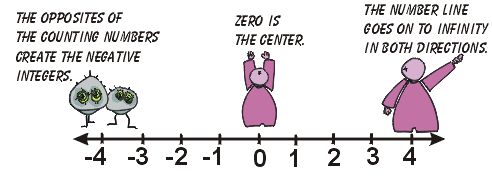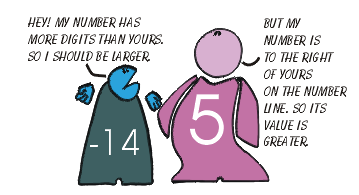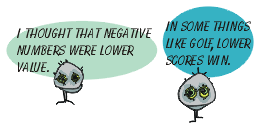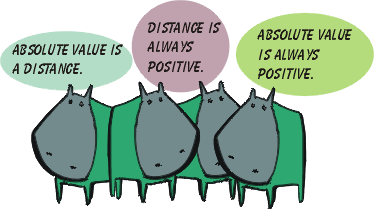##### The set of integers is composed of the counting (natural ) numbers, their opposites, and zero. Begining with zero, numbers increase in value to the right (0, 1, 2, 3, …) and decrease in value to the left (…-3, -2, -1, 0). When comparing numbers, the order in which they are placed on the number line will determine if it is greater than or less than other numbers.If a number is to the left of a number on the number line, it is less than the other number. If it is to the right, then it is greater than that number.

 Example: If the lowest score wins, order the following golf scores from best to worst: Tigre Madera –4, Jack Nickles +1, Nick Cost –2, Freddy Pairs –5, John Weekly +3 Answer: -5, -4, -2, +1, +3The Picksburg running back, nicknamed "The Trolley," had five carries with the following results: +18, -3, +4, 0, -1. List these yardage figures from best to worst.##Absolute value is the distance a number is from zero on a number line. We use |n | to indicate absolute value. |-n| = |n| =n; where n is an integer. Example: |-7| = 7 Example: Jack Nickles is –4 after 36 holes of golf. How many strokes away from even par is he? (Par for a course is a score of zero strokes above or below.) Answer: 4Example: |7 + 3| - |-9| = 10 – 9 = 1  writer: Kevin Gallagher/mC

Integer Main | Interactive Adventure with Deep Sea Carl

 art: Zach Sterba/mC writer: Kevin Gallagher/mC